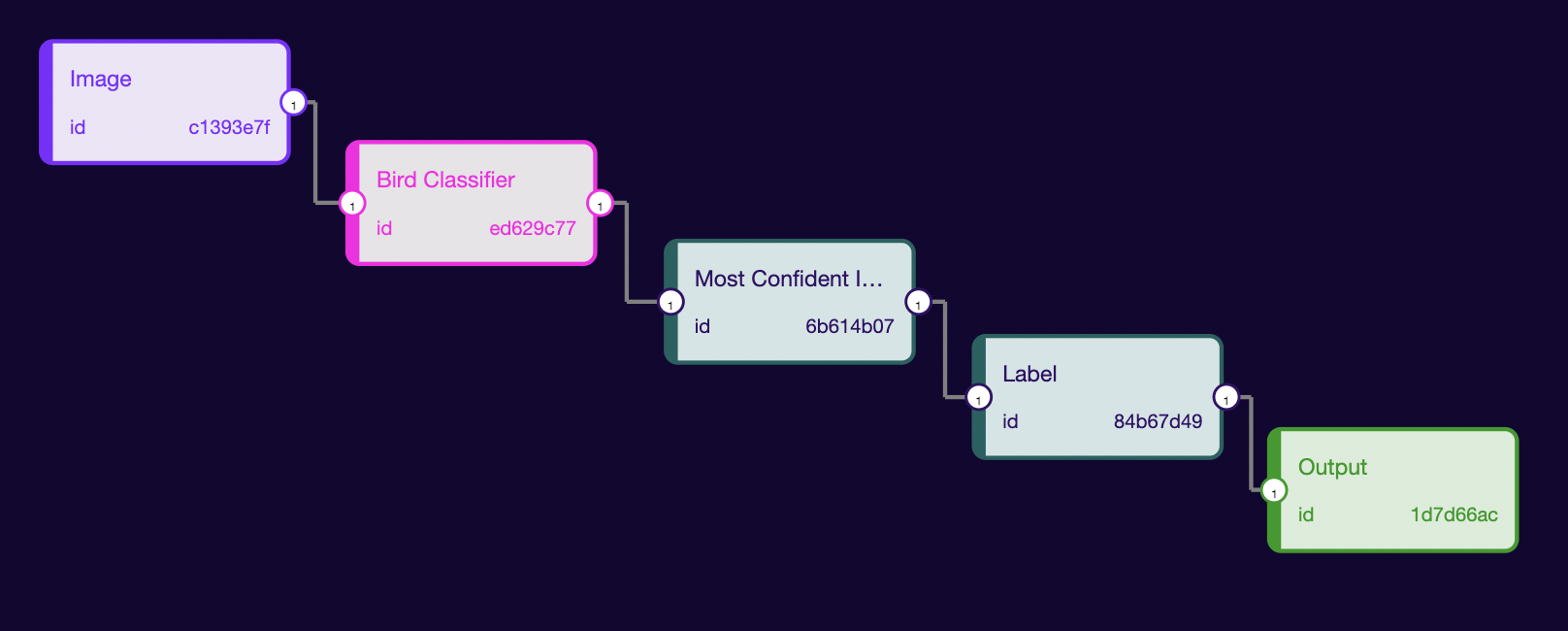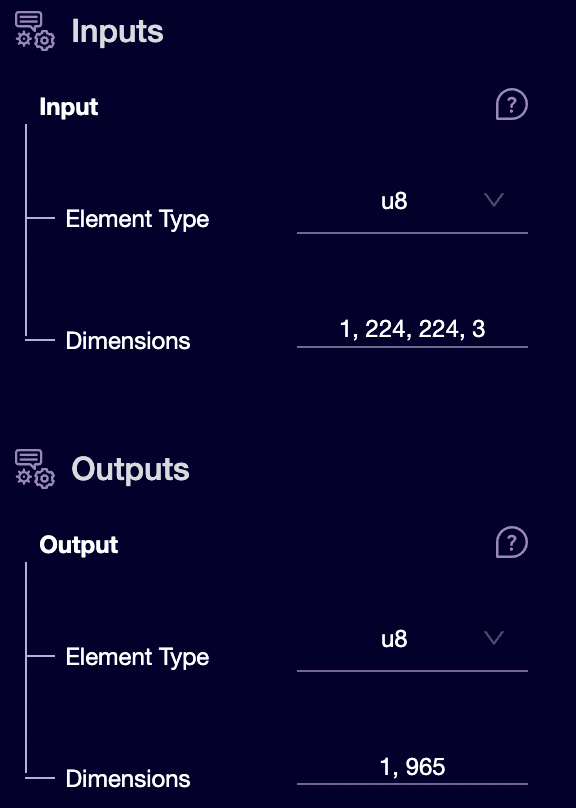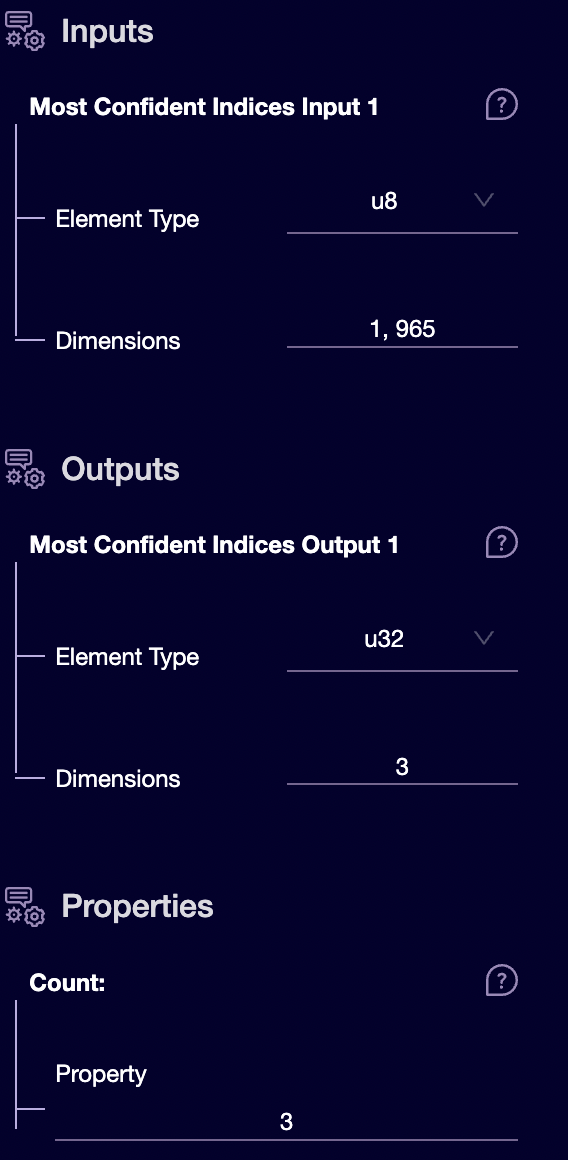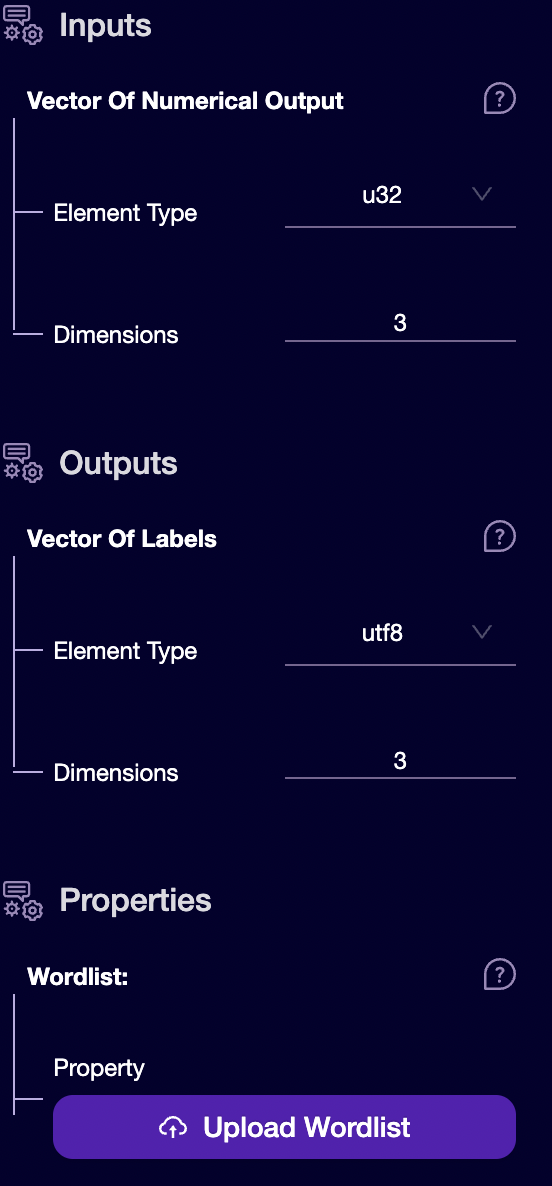# Bird Classifier

An image classification model that classifies birds from images.The first step of creating a Rune is finding (or training) a Machine Learning Model that matches your application. Here, we have decided to choose the Bird Classification model. We will start by knowing the model input/output information. So, click on the model node present inside the studio with the name of Bird Classification. It will show the Input/Output information. This information will be used to build the ML Pipeline.Comparing input with the image format: `[batch_size, height, width, channels]`, we can see the model will take a `224 x 224` RGB image (because the channel is 3). The input image type is `u8`, i.e., all the values will lie between `[0, 255]`. The output is an array of size `[1, 965]`. The model will return a list with scores for `965` labels. We will have to find the top `3` score and their associated labels. Now, we have understood how the data will flow, let’s start with creating the ML pipeline.

1. Drag an Image from the Inputs: We will set the output type to `u8` and dimensions to `[1, 224, 224, 3]` because, as we saw above, this is the format needed by our model. The IMAGE capability takes the following arguments:
• width - the image's width in pixels
• height - the image's height in pixels
• pixel-format - the format used by the pixels. Possible values are:
• @PixelFormat::Grayscale
• @PixelFormat::RGB
2. Drag the Bird Classifier model from the left side panel.
3. We have got the output score for all three classes from our model. We want to find out which `3` classes with the highest score so that later we can print that class name as output. To get the index of class with the highest confidence value, we will use most_confident_indices proc-block (a proc block which, when given a list of confidences, will return the indices of the top N most confident values). So, drag the most_confident_indices proc-block from the left side pan.Set input dimension same as output dimensions of the model `(1,965)`. We want the three most confident values in the output, so set it to `3`. You will have to set the count the same as the output dimensions.
4. Till now, we have got an index of the most confident index. Next, we would like to assign a label to this index. We will follow the above approach and connect the output of the most_confident_indices node to the input of our label node. Fill the Input values the same as the output dimension of most_confident_indices. We will get a string as output, so we will have to set the output type to `utf8` and dimensions to `3`. In Properties, we will upload the labels of our model. You can find the label file here.5. Finally, connect the output of the label proc-block node to the input of the `Output` node